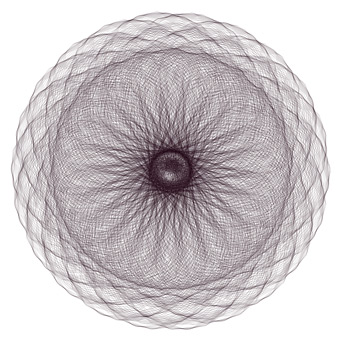## hypotrochoidWe start projects in english with this amazing animated parametric curve. Just run the code, it will use random values of parameters that you can then adjust. If you ask for our favorite settings, here you have:

r_1 = 26; r_2 = 156; r_3 = 25;

r_1 = 192; r_2 = 48; r_3 = 72;

Try other settings. And then change the number or r's inside the code:

"nr := 3 ;or 4, 5, ...

How the curve is created? There are multiple circles rotating within each other. The last circle has an arm with a pen. The simplest case is below:But the curve quickly becomes complicated when more circles are added. Also the arm can be different than the circle radius:In the program, to animate the curve, the arm is slowly changing its lentgh.